Main Content

# infer

Infer conditional variances of conditional variance models

## Syntax

``V = infer(Mdl,Y)``
``````[V,logL] = infer(Mdl,Y)``````
``````[V,logL] = infer(Mdl,Y,Name,Value)``````

## Description

example

````V = infer(Mdl,Y)` infers the conditional variances of the fully specified, univariate conditional variance model `Mdl` fit to the response data `Y`. `Mdl` can be a `garch`, `egarch`, or `gjr` model.```

example

``````[V,logL] = infer(Mdl,Y)``` additionally returns the loglikelihood objective function values.```

example

``````[V,logL] = infer(Mdl,Y,Name,Value)``` infers the conditional variances of `Mdl` with additional options specified by one or more `Name,Value` pair arguments. For example, you can specify presample innovations or conditional variances.```

## Examples

collapse all

Infer conditional variances from a GARCH(1,1) model with known coefficients. When you use, and then do not use presample data, compare the results from `infer`.

Specify a GARCH(1,1) model with known parameters. Simulate 101 conditional variances and responses (innovations) from the model. Set aside the first observation from each series to use as presample data.

```Mdl = garch('Constant',0.01,'GARCH',0.8,'ARCH',0.15); rng default; % For reproducibility [vS,yS] = simulate(Mdl,101); y0 = yS(1); v0 = vS(1); y = yS(2:end); v = vS(2:end); figure subplot(2,1,1) plot(v) title('Conditional Variances') subplot(2,1,2) plot(y) title('Innovations') ```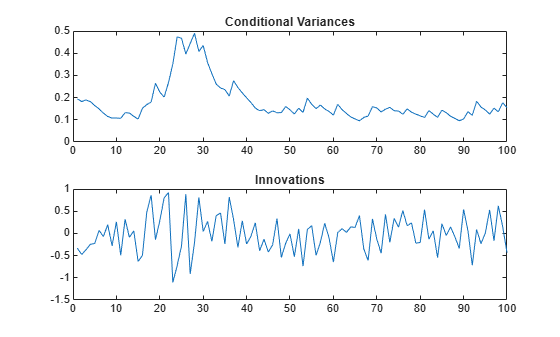Infer the conditional variances of `y` without using presample data. Compare them to the known (simulated) conditional variances.

```vI = infer(Mdl,y); figure plot(1:100,v,'r','LineWidth',2) hold on plot(1:100,vI,'k:','LineWidth',1.5) legend('Simulated','Inferred','Location','NorthEast') title('Inferred Conditional Variances - No Presamples') hold off ```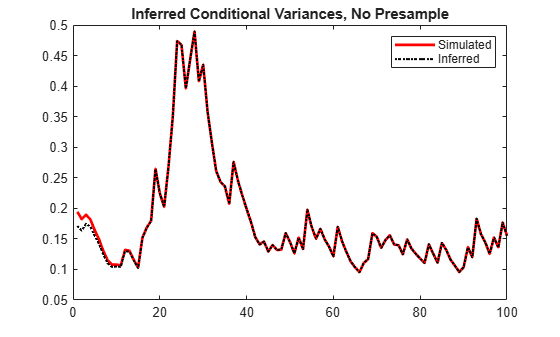Notice the transient response (discrepancy) in the early time periods due to the absence of presample data.

Infer conditional variances using the set-aside presample innovation, `y0`. Compare them to the known (simulated) conditional variances.

```vE = infer(Mdl,y,'E0',y0); figure plot(1:100,v,'r','LineWidth',2) hold on plot(1:100,vE,'k:','LineWidth',1.5) legend('Simulated','Inferred','Location','NorthEast') title('Inferred Conditional Variances - Presample E') hold off ```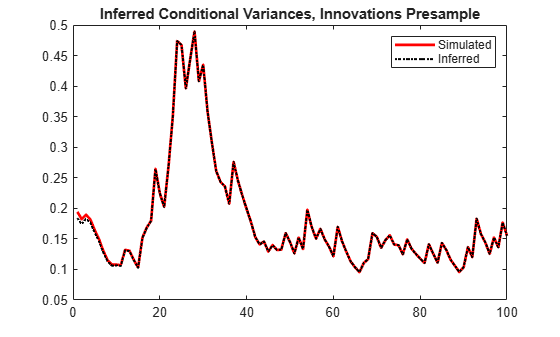There is a slightly reduced transient response in the early time periods.

Infer conditional variances using the set-aside presample conditional variance, `v0`. Compare them to the known (simulated) conditional variances.

```vO = infer(Mdl,y,'V0',v0); figure plot(v) plot(1:100,v,'r','LineWidth',2) hold on plot(1:100,vO,'k:','LineWidth',1.5) legend('Simulated','Inferred','Location','NorthEast') title('Inferred Conditional Variances - Presample V') hold off ```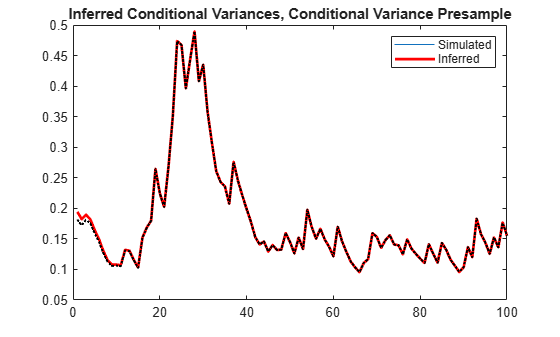There is a much smaller transient response in the early time periods.

Infer conditional variances using both the presample innovation and conditional variance. Compare them to the known (simulated) conditional variances.

```vEO = infer(Mdl,y,'E0',y0,'V0',v0); figure plot(v) plot(1:100,v,'r','LineWidth',2) hold on plot(1:100,vEO,'k:','LineWidth',1.5) legend('Simulated','Inferred','Location','NorthEast') title('Inferred Conditional Variances - Presamples') hold off ```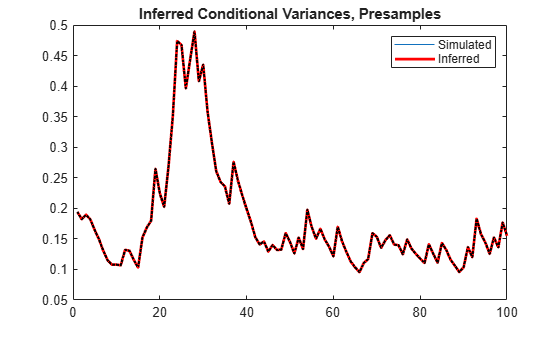When you use sufficient presample innovations and conditional variances, the inferred conditional variances are exact (there is no transient response).

Infer conditional variances from an EGARCH(1,1) model with known coefficients. When you use, and then do not use presample data, compare the results from `infer`.

Specify an EGARCH(1,1) model with known parameters. Simulate 101 conditional variances and responses (innovations) from the model. Set aside the first observation from each series to use as presample data.

```Mdl = egarch('Constant',0.001,'GARCH',0.8,... 'ARCH',0.15,'Leverage',-0.1); rng default; % For reproducibility [vS,yS] = simulate(Mdl,101); y0 = yS(1); v0 = vS(1); y = yS(2:end); v = vS(2:end); figure subplot(2,1,1) plot(v) title('Conditional Variances') subplot(2,1,2) plot(y) title('Innovations') ```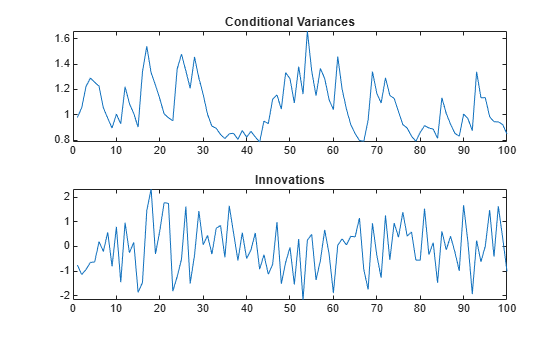Infer the conditional variances of `y` without using any presample data. Compare them to the known (simulated) conditional variances.

```vI = infer(Mdl,y); figure plot(1:100,v,'r','LineWidth',2) hold on plot(1:100,vI,'k:','LineWidth',1.5) legend('Simulated','Inferred','Location','NorthEast') title('Inferred Conditional Variances - No Presamples') hold off ```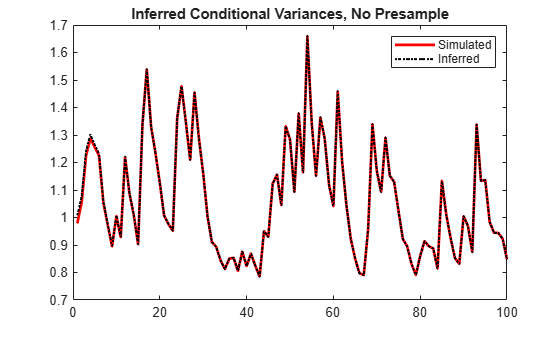Notice the transient response (discrepancy) in the early time periods due to the absence of presample data.

Infer conditional variances using the set-aside presample innovation, `y0`. Compare them to the known (simulated) conditional variances.

```vE = infer(Mdl,y,'E0',y0); figure plot(1:100,v,'r','LineWidth',2) hold on plot(1:100,vE,'k:','LineWidth',1.5) legend('Simulated','Inferred','Location','NorthEast') title('Inferred Conditional Variances - Presample E') hold off ```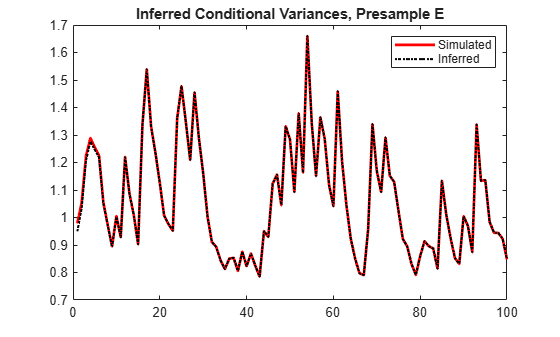There is a slightly reduced transient response in the early time periods.

Infer conditional variances using the set-aside presample variance, `v0`. Compare them to the known (simulated) conditional variances.

```vO = infer(Mdl,y,'V0',v0); figure plot(v) plot(1:100,v,'r','LineWidth',2) hold on plot(1:100,vO,'k:','LineWidth',1.5) legend('Simulated','Inferred','Location','NorthEast') title('Inferred Conditional Variances - Presample V') hold off ```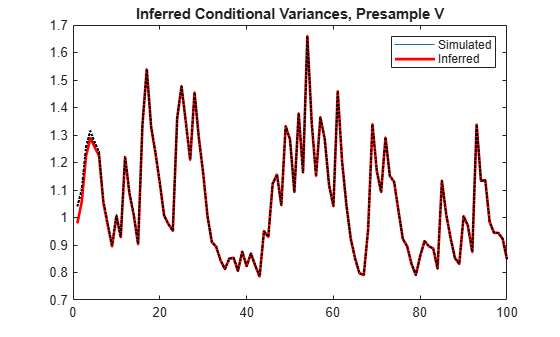The transient response is almost eliminated.

Infer conditional variances using both the presample innovation and conditional variance. Compare them to the known (simulated) conditional variances.

```vEO = infer(Mdl,y,'E0',y0,'V0',v0); figure plot(v) plot(1:100,v,'r','LineWidth',2) hold on plot(1:100,vEO,'k:','LineWidth',1.5) legend('Simulated','Inferred','Location','NorthEast') title('Inferred Conditional Variances - Presamples') hold off ```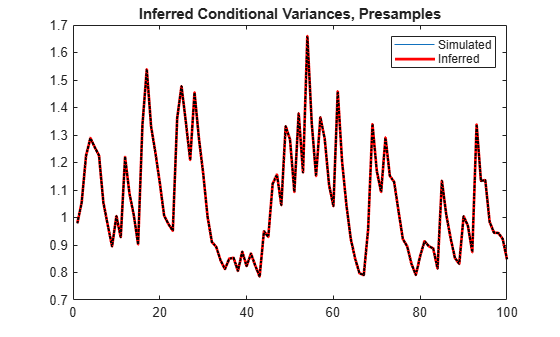When you use sufficient presample innovations and conditional variances, the inferred conditional variances are exact (there is no transient response).

Infer conditional variances from a GJR(1,1) model with known coefficients. When you use, and then do not use presample data, compare the results from `infer`.

Specify a GJR(1,1) model with known parameters. Simulate 101 conditional variances and responses (innovations) from the model. Set aside the first observation from each series to use as presample data.

```Mdl = gjr('Constant',0.01,'GARCH',0.8,'ARCH',0.14,... 'Leverage',0.1); rng default; % For reproducibility [vS,yS] = simulate(Mdl,101); y0 = yS(1); v0 = vS(1); y = yS(2:end); v = vS(2:end); figure subplot(2,1,1) plot(v) title('Conditional Variances') subplot(2,1,2) plot(y) title('Innovations') ```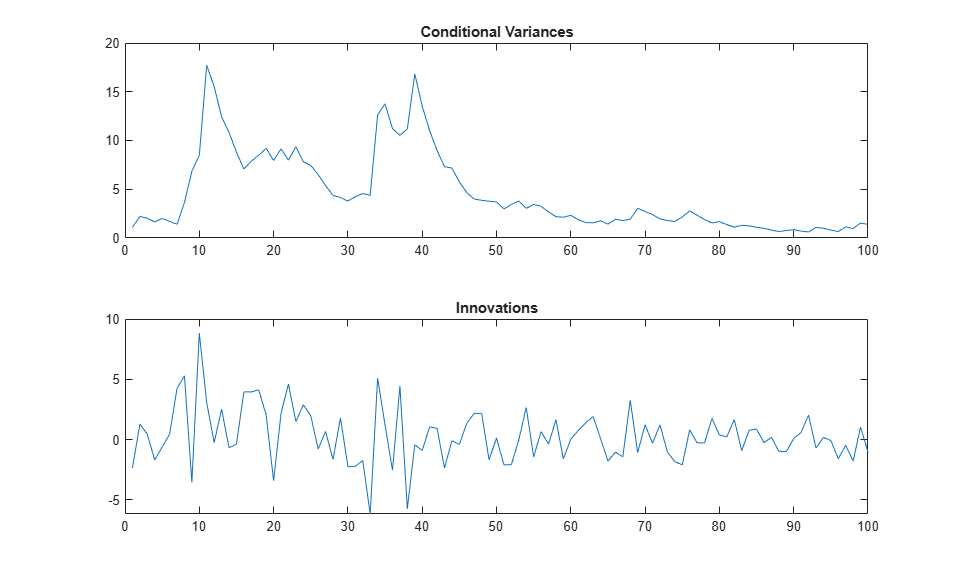Infer the conditional variances of `y` without using any presample data. Compare them to the known (simulated) conditional variances.

```vI = infer(Mdl,y); figure plot(1:100,v,'r','LineWidth',2) hold on plot(1:100,vI,'k:','LineWidth',1.5) legend('Simulated','Inferred','Location','NorthEast') title('Inferred Conditional Variances - No Presamples') hold off ```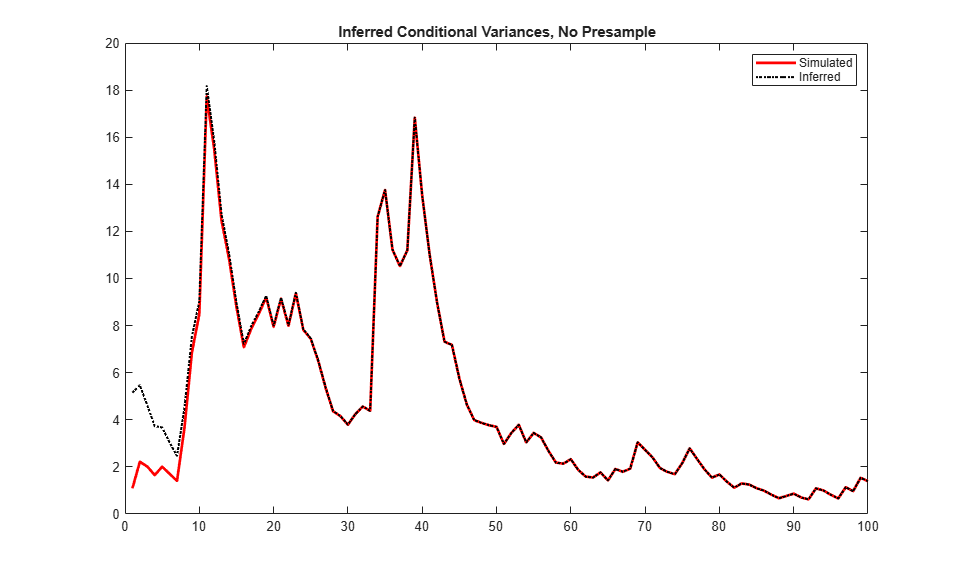Notice the transient response (discrepancy) in the early time periods due to the absence of presample data.

Infer conditional variances using the set-aside presample innovation, `y0`. Compare them to the known (simulated) conditional variances.

```vE = infer(Mdl,y,'E0',y0); figure plot(1:100,v,'r','LineWidth',2) hold on plot(1:100,vE,'k:','LineWidth',1.5) legend('Simulated','Inferred','Location','NorthEast') title('Inferred Conditional Variances - Presample E') hold off ```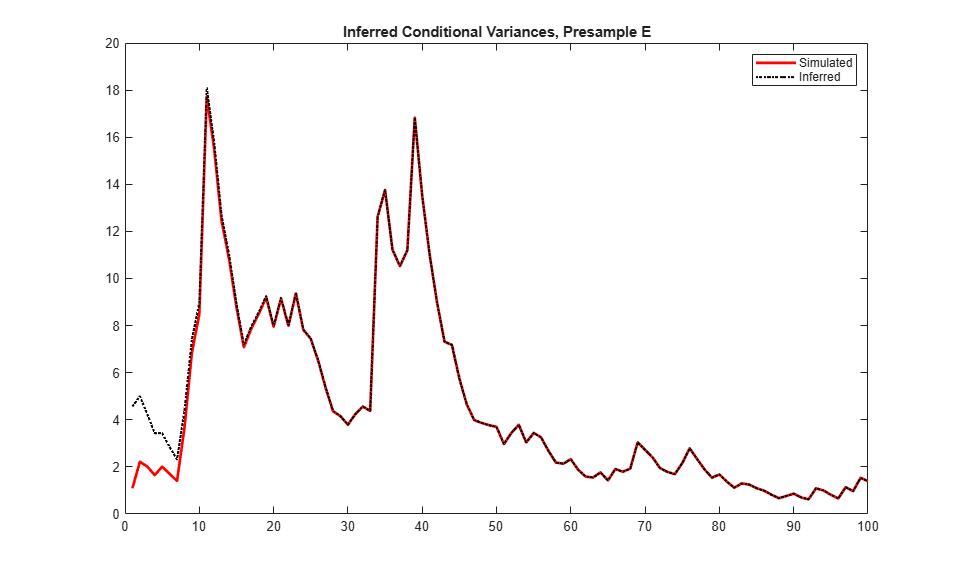There is a slightly reduced transient response in the early time periods.

Infer conditional variances using the set-aside presample conditional variance, `vO`. Compare them to the known (simulated) conditional variances.

```vO = infer(Mdl,y,'V0',v0); figure plot(v) plot(1:100,v,'r','LineWidth',2) hold on plot(1:100,vO,'k:','LineWidth',1.5) legend('Simulated','Inferred','Location','NorthEast') title('Inferred Conditional Variances - Presample V') hold off ```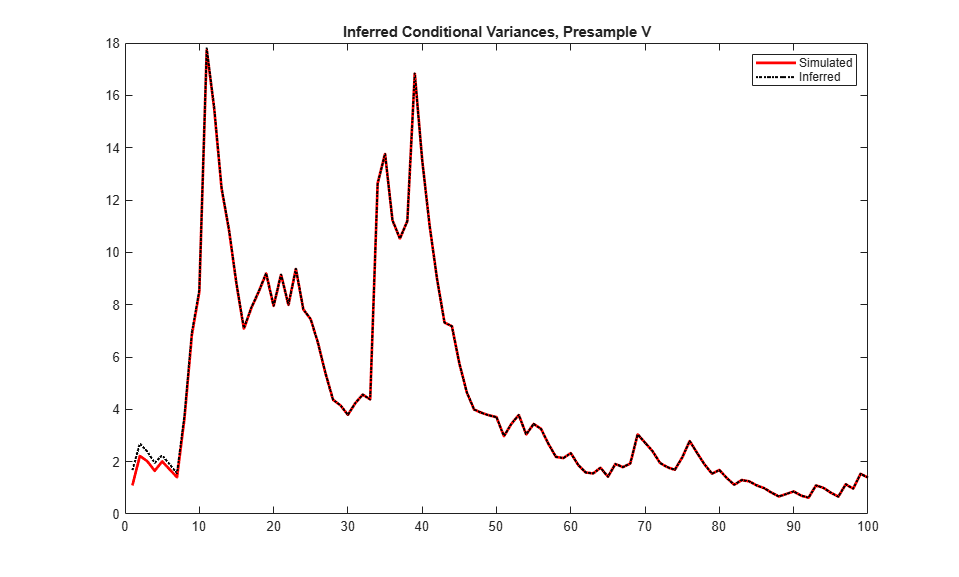There is a much smaller transient response in the early time periods.

Infer conditional variances using both the presample innovation and conditional variance. Compare them to the known (simulated) conditional variances.

```vEO = infer(Mdl,y,'E0',y0,'V0',v0); figure plot(v) plot(1:100,v,'r','LineWidth',2) hold on plot(1:100,vEO,'k:','LineWidth',1.5) legend('Simulated','Inferred','Location','NorthEast') title('Inferred Conditional Variances - Presamples') hold off ```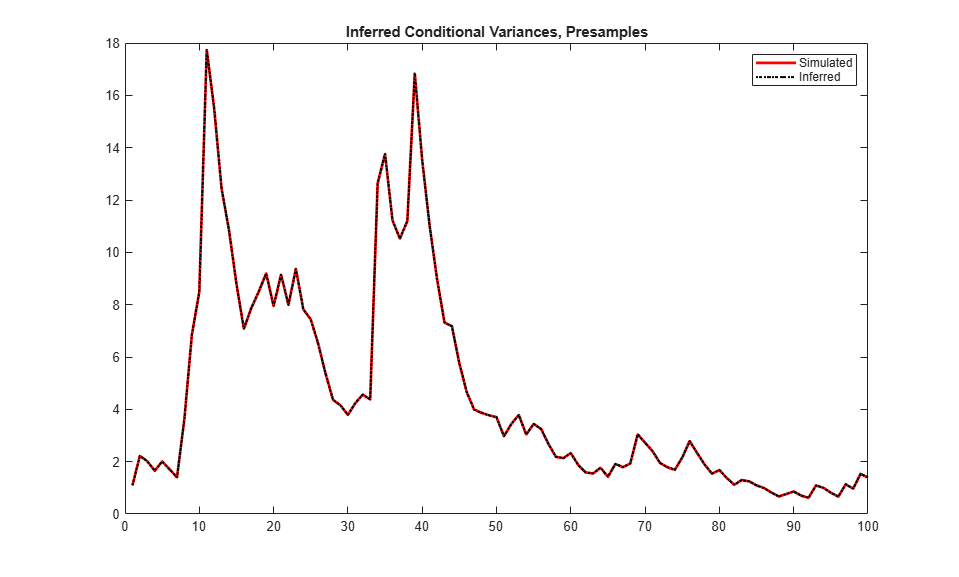When you use sufficient presample innovations and conditional variances, the inferred conditional variances are exact (there is no transient response).

Infer the loglikelihood objective function values for an EGARCH(1,1) and EGARCH(2,1) model fit to NASDAQ Composite Index returns. To identify which model is the more parsimonious, adequate fit, conduct a likelihood ratio test.

Load the NASDAQ data included with the toolbox, and convert the index to returns. Set aside the first two observations to use as presample data.

```load Data_EquityIdx nasdaq = DataTable.NASDAQ; r = price2ret(nasdaq); r0 = r(1:2); rn = r(3:end);```

Fit an EGARCH(1,1) model to the returns, and infer the loglikelihood objective function value.

```Mdl1 = egarch(1,1); EstMdl1 = estimate(Mdl1,rn,'E0',r0);```
``` EGARCH(1,1) Conditional Variance Model (Gaussian Distribution): Value StandardError TStatistic PValue _________ _____________ __________ __________ Constant -0.13518 0.022134 -6.1074 1.0129e-09 GARCH{1} 0.98386 0.0024268 405.41 0 ARCH{1} 0.19997 0.013993 14.29 2.5182e-46 Leverage{1} -0.060244 0.0056558 -10.652 1.7129e-26 ```
`[~,logL1] = infer(EstMdl1,rn,'E0',r0);`

Fit an EGARCH(2,1) model to the returns, and infer the loglikelihood objective function value.

```Mdl2 = egarch(2,1); EstMdl2 = estimate(Mdl2,rn,'E0',r0);```
``` EGARCH(2,1) Conditional Variance Model (Gaussian Distribution): Value StandardError TStatistic PValue _________ _____________ __________ __________ Constant -0.1456 0.028436 -5.1202 3.0524e-07 GARCH{1} 0.85307 0.14018 6.0854 1.1618e-09 GARCH{2} 0.12952 0.13838 0.93597 0.34929 ARCH{1} 0.21969 0.029465 7.456 8.9205e-14 Leverage{1} -0.067936 0.01088 -6.2444 4.2552e-10 ```
`[~,logL2] = infer(EstMdl2,rn,'E0',r0);`

Conduct a likelihood ratio test, with the more parsimonious EGARCH(1,1) model as the null model, and the EGARCH(2,1) model as the alternative. The degree of freedom for the test is 1, because the EGARCH(2,1) model has one more parameter than the EGARCH(1,1) model (an additional GARCH term).

`[h,p] = lratiotest(logL2,logL1,1)`
```h = logical 0 ```
```p = 0.2256 ```

The null hypothesis is not rejected (`h = 0`). At the 0.05 significance level, the EGARCH(1,1) model is not rejected in favor of the EGARCH(2,1) model.

A GARCH(P, Q) model is nested within a GJR(P, Q) model. Therefore, you can perform a likelihood ratio test to compare GARCH(P, Q) and GJR(P, Q) model fits.

Infer the loglikelihood objective function values for a GARCH(1,1) and GJR(1,1) model fit to NASDAQ Composite Index returns. Conduct a likelihood ratio test to identify which model is the more parsimonious, adequate fit.

Load the NASDAQ data included with the toolbox, and convert the index to returns. Set aside the first two observations to use as presample data.

```load Data_EquityIdx nasdaq = DataTable.NASDAQ; r = price2ret(nasdaq); r0 = r(1:2); rn = r(3:end);```

Fit a GARCH(1,1) model to the returns, and infer the loglikelihood objective function value.

```Mdl1 = garch(1,1); EstMdl1 = estimate(Mdl1,rn,'E0',r0);```
``` GARCH(1,1) Conditional Variance Model (Gaussian Distribution): Value StandardError TStatistic PValue _________ _____________ __________ __________ Constant 2.005e-06 5.4298e-07 3.6926 0.00022197 GARCH{1} 0.88333 0.0084536 104.49 0 ARCH{1} 0.10924 0.0076666 14.249 4.5737e-46 ```
`[~,logL1] = infer(EstMdl1,rn,'E0',r0);`

Fit a GJR(1,1) model to the returns, and infer the loglikelihood objective function value.

```Mdl2 = gjr(1,1); EstMdl2 = estimate(Mdl2,rn,'E0',r0);```
``` GJR(1,1) Conditional Variance Model (Gaussian Distribution): Value StandardError TStatistic PValue __________ _____________ __________ __________ Constant 2.4752e-06 5.6983e-07 4.3438 1.4006e-05 GARCH{1} 0.88102 0.0095104 92.637 0 ARCH{1} 0.064015 0.0091849 6.9696 3.1787e-12 Leverage{1} 0.089297 0.0099211 9.0007 2.2426e-19 ```
`[~,logL2] = infer(EstMdl2,rn,'E0',r0);`

Conduct a likelihood ratio test, with the more parsimonious GARCH(1,1) model as the null model, and the GJR(1,1) model as the alternative. The degree of freedom for the test is 1, because the GJR(1,1) model has one more parameter than the GARCH(1,1) model (a leverage term).

`[h,p] = lratiotest(logL2,logL1,1)`
```h = logical 1 ```
```p = 4.5816e-10 ```

The null hypothesis is rejected (`h = 1`). At the 0.05 significance level, the GARCH(1,1) model is rejected in favor of the GJR(1,1) model.

## Input Arguments

collapse all

Conditional variance model without any unknown parameters, specified as a `garch`, `egarch`, or `gjr` model object.

`Mdl` cannot contain any properties that have `NaN` value.

Response data, specified as a numeric column vector or matrix.

As a column vector, `Y` represents a single path of the underlying series.

As a matrix, the rows of `Y` correspond to periods and the columns correspond to separate paths. The observations across any row occur simultaneously.

`infer` infers the conditional variances of `Y`. `Y` usually represents an innovation series with mean 0 and variances characterized by `Mdl`. It is the continuation of the presample innovation series `E0`. `Y` can also represent a time series of innovations with mean 0 plus an offset. If `Mdl` has a nonzero offset, then the software stores its value in the `Offset` property (`Mdl.Offset`).

`infer` assumes that observations across any row occur simultaneously.

The last observation of any series is the latest observation.

Note

`NaN`s indicate missing values. `infer` removes missing values. `infer` uses list-wise deletion to remove any `NaN`s. Removing `NaN`s in the data reduces the sample size. Removing missing values, can also create irregular time series.

### Name-Value Pair Arguments

Specify optional comma-separated pairs of `Name,Value` arguments. `Name` is the argument name and `Value` is the corresponding value. `Name` must appear inside quotes. You can specify several name and value pair arguments in any order as `Name1,Value1,...,NameN,ValueN`.

Example: `'E0',[1 1;0.5 0.5],'V0',[1 0.5;1 0.5]` specifies two equivalent presample paths of innovations and two, different presample paths of conditional variances.

Presample innovations, specified as the comma-separated pair consisting of `'E0'` and a numeric column vector or matrix. The presample innovations provide initial values for the innovations process of the conditional variance model `Mdl`, and derive from a distribution with mean 0.

`E0` must contain at least `Mdl.Q` elements or rows. If `E0` contains extra rows, then `infer` uses the latest `Mdl.Q` only.

The last element or row contains the latest presample innovation.

• If `E0` is a column vector, it represents a single path of the underlying innovation series. `infer` applies `E0` to each inferred path.

• If `E0` is a matrix, then each column represents a presample path of the underlying innovation series. `E0` must have at least as many columns as `Y`. If `E0` has more columns than necessary, `infer` uses the first `size(Y,2)` columns only.

The defaults are:

• For GARCH(P,Q) and GJR(P,Q) models, `infer` sets any necessary presample innovations to the square root of the average squared value of the offset-adjusted response series `Y`.

For EGARCH(P,Q) models, `infer` sets any necessary presample innovations to zero.

Example: `'E0',[1 1;0.5 0.5]`

Data Types: `double`

Presample conditional variances, specified as the comma-separated pair consisting of `'V0'` and a numeric column vector or matrix with positive entries. `V0` provides initial values for the conditional variances in the model.

• If `V0` is a column vector, then `infer` applies it to each output path.

• If `V0` is a matrix, then each column represents a presample path of conditional variances. `V0` must have at least as many columns as `Y`. If `V0` has more columns than required, `infer` uses the first `size(Y,2)` columns only.

• For GARCH(P,Q) and GJR(P,Q) models, `V0` must have at least `Mdl.P` rows (or elements) to initialize the variance equation.

• For EGARCH(P,Q) models, `V0` must have at least `max(Mdl.P,Mdl.Q)` rows to initialize the variance equation.

If the number of rows in `V0` exceeds the necessary number, then `infer` uses the latest, required number of observations only.

The last element row contains the latest observation.

By default, `infer` sets any necessary observations to the average squared value of the offset-adjusted response series `Y`.

Example: `'V0',[1 0.5;1 0.5]`

Data Types: `double`

Notes:

• `NaN`s indicate missing values. `infer` removes missing values. The software merges the presample data (`E0` and `V0`) separately from the input response data (`Y`), and then uses list-wise deletion to remove any rows containing at least one `NaN`. Removing `NaN`s in the data reduces the sample size. Removing missing values can also create irregular time series.

• `infer` assumes that you synchronize presample data such that the latest observation of each presample series occurs simultaneously.

• If you do not specify `E0` and `V0`, then `infer` derives the necessary presample observations from the unconditional, or long-run, variance of the offset-adjusted response process.

• For all conditional variance models, `V0` is the sample average of the squared disturbances of the offset-adjusted response data `Y`.

• For GARCH(P,Q) and GJR(P,Q) models, `E0` is the square root of the average squared value of the offset-adjusted response series `Y`.

• For EGARCH(P,Q) models, `E0` is `0`.

These specifications minimize initial transient effects.

## Output Arguments

collapse all

Conditional variances inferred from the response data `Y`, returned as a numeric column vector or matrix.

The dimensions of `V` and `Y` are equivalent. If `Y` is a matrix, then the columns of `V` are the inferred conditional variance paths corresponding to the columns of `Y`.

Rows of `V` are periods corresponding to the periodicity of `Y`.

Loglikelihood objective function values associated with the model `Mdl`, returned as a scalar or numeric vector.

If `Y` is a vector, then `logL` is a scalar. Otherwise, `logL` is vector of length `size(Y,2)`, and each element is the loglikelihood of the corresponding column (or path) in `Y`.

Data Types: `double`

 Bollerslev, T. “Generalized Autoregressive Conditional Heteroskedasticity.” Journal of Econometrics. Vol. 31, 1986, pp. 307–327.

 Bollerslev, T. “A Conditionally Heteroskedastic Time Series Model for Speculative Prices and Rates of Return.” The Review of Economics and Statistics. Vol. 69, 1987, pp. 542–547.

 Box, G. E. P., G. M. Jenkins, and G. C. Reinsel. Time Series Analysis: Forecasting and Control. 3rd ed. Englewood Cliffs, NJ: Prentice Hall, 1994.

 Enders, W. Applied Econometric Time Series. Hoboken, NJ: John Wiley & Sons, 1995.

 Engle, R. F. “Autoregressive Conditional Heteroskedasticity with Estimates of the Variance of United Kingdom Inflation.” Econometrica. Vol. 50, 1982, pp. 987–1007.

 Glosten, L. R., R. Jagannathan, and D. E. Runkle. “On the Relation between the Expected Value and the Volatility of the Nominal Excess Return on Stocks.” The Journal of Finance. Vol. 48, No. 5, 1993, pp. 1779–1801.

 Hamilton, J. D. Time Series Analysis. Princeton, NJ: Princeton University Press, 1994.

Download ebook